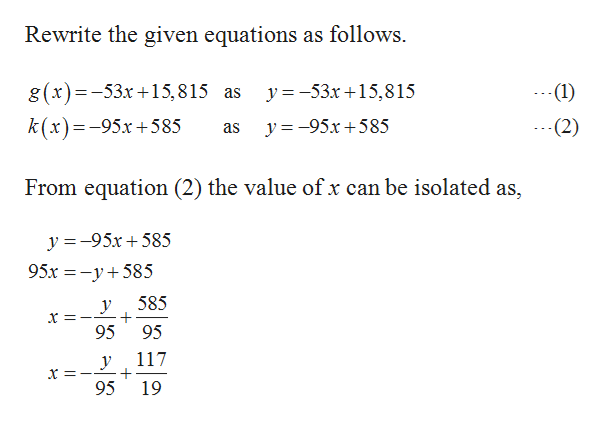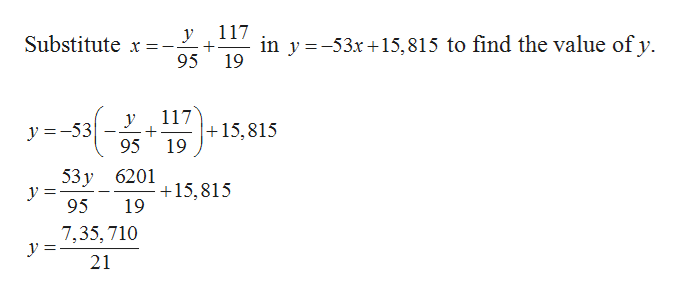# Use algebra to find the point at which the line g(x)=−53x+15815 intersects the line k(x)=−95x+585Write the values of x and y as reduced fractions or integers.x=y=

Question

Use algebra to find the point at which the line g(x)=−53x+15815 intersects the line k(x)=−95x+585

Write the values of x and y as reduced fractions or integers.

x=
y=

check_circleExpert Solution
Step 1help_outlineImage TranscriptioncloseRewrite the given equations as follows g(x)=-53x15,815 as -(1) y -53x15,815 k(x)=-95x+585 y-95x 585 -(2) as From equation (2) the value of x can be isolated as, y =-95x585 95x -y585 585 у 95 95 117 у + 19 95 fullscreen
Step 2help_outlineImage Transcriptionclose117 у in y -53x15,815 to find the value of y 19 Substitute x=-. 95 117 15,815 19 у y =-53 95 53 у уУ 3 95 6201 +15,815 19 7,35, 710 у 3 21 fullscreen

### Want to see the full answer?

See Solution

#### Want to see this answer and more?

Solutions are written by subject experts who are available 24/7. Questions are typically answered within 1 hour*

See Solution
*Response times may vary by subject and question
Tagged in

### Other